# Coulomb's Law and Electric Fields

Coulomb's Law

Electric charges attract and repel by exerting forces on each other. Coulomb's law describes this force. It is the basic law of interaction between electric charges. Specifically, Coulomb's law deals with point charges. Point charges can be protons, electrons, or other basic particles of matter. Additionally, any objects can be treated as point charges, as long as the objects are very small in comparison to the distance between them. In words, Coulomb's law is: The magnitude of the electric force between to point charges is proportional to the magnitude of the charges, and inversely proportional to the distance between them.

For an electrostatic force of magnitude F, Coulomb's law is expressed with the formula,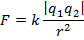In this formula, q1 is the charge of point charge 1, and q2 is the charge of point charge 2. The distance between these point charges is r. The Coulomb constant k defines the proportionality, and will be discussed in detail below. The direction of the force is a vector along the line that joins the two charges. The forces on the two point charges form an action-reaction pair, according to Newton's Third Law. This means that the magnitude of the force is the same on both point charges, and that the directions of the forces are opposite. If the two charges have the same sign (both are positive or both are negative), then the forces are repulsive, and point away from the other charged object. If the two charges have the opposite signs, then the forces are attractive, and point toward the other charged object. The sign of the vector force depends on whether the force is attractive or repulsive. The unit vector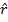can be used to indicate a direction that follows the line between the charges. The vector force can be written,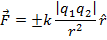In SI units, the unit of electric charge is called the Coulomb. It is one of the fundamental units of the SI system. The Coulomb unit is represented with the letter C. In the above formula for Coulomb's law, the charge values q1 and q2 are expressed in Coulombs, with either a positive or negative sign. In SI units, the value of r is expressed in meters (m) and the result is a force F expressed in Newtons (N).

The constant k is Coulomb's law has a value that was determined experimentally to be,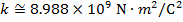The constant k can also be written in terms of another constant, called the permittivity of free space. The symbol used for this constant is the Greek letter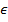("epsilon") with a subscript zero: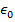. This is pronounced "epsilon-nought". The value ofis,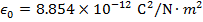The relation between k andis,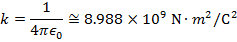This means that Coulomb's law is often written,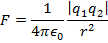The two versions of the formula are equivalent.

Charge can only be subdivided in to multiples of the electron or proton charge. Any value of charge must be a multiple of this value. The smallest possible charge magnitude is labeled e. Expressed in Coulombs, the value of e is,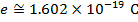The charge of a single proton is therefore,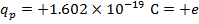The charge of a single electron is therefore,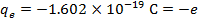For simplicity, the charge of objects are often written as multiples of e. For example, the charge of a group of 10 protons and 8 electrons together would be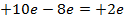.

Superposition of Forces

Coulomb's law defines the forces that act between two point charges. When more point charges are introduced, the forces on each charge sum together. This is called the superposition of forces. When two or more charges each exert a force on another point charge, the total force on that charge is the vector sum of the forces exerted by the other charges.

For example, the force on point charge 1 exerted by point charges 2, 3, and so on is,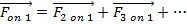Electric Fields

Every charged object emits an electric field. This electric field is the origin of the electric force that other charged particles experience. The electric field of a charge exists everywhere, but its strength decreases with distance squared. In SI units, the electric field unit is Newtons per Coulomb,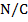.

The electric field of a charged object can be found using a test charge. A test charge is a small charge that can be placed at various positions to map an electric field. The test charge is labeled q0. If a test charge placed at a certain position experiences an electrostatic force, then an electric field exists at that position. The electrostatic force at the position of the test charge is labeled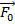.

Electrostatic force is a vector quantity, and so is electric field. The electric field at a certain position is equal to the electrostatic forceat that position, divided by the test charge q0,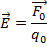If the electric field at a certain position is known, then this formula can be rearranged to solve for the electrostatic force on the test charge q0,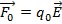The sign of the test charge determines the relationship between the electric field and electrostatic force directions. If the test charge is positive, then the force and field vectors have the same direction. If the test charge is negative, then the force and field vectors have opposite directions.

If the source of the electric field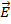is a point charge q, then the electrostatic force is between this point charge and the test charge q0. The position of the point charge q is called the source point, and the position of the test charge q0 is called the field point. The distance between these points is r, and the unit vector that points from the source point toward the field point is. The magnitude of the force at the field point is,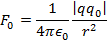From this formula, it is possible to solve for the magnitude of the electric field,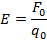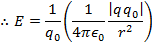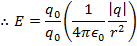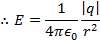The vector direction of the electric field is defined such that the vector always points away from positive charges. For that reason, the direction is always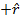when q is positive, and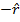when q is negative. Thus, the vector formula for the electric field is,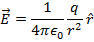Electric field vectors point away from positive sources, and toward negative sources.

Superposition of Fields

When there is more than a single point source of an electric field, the total electric field is the vector sum of the charges that contribute to it. This is called the superposition of fields. If the charges are labeled 1, 2, 3, and so on, the total electric field is,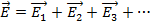From this formula, the total force on the test charge q0 can be found,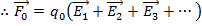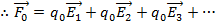This formula shows the connection between the superposition of fields and the superposition of forces.

Electric Field Lines

A map of the vectors formed by an electric field can be found by moving a test charge q0 to many positions around the sources. This map forms a vector field. The field vectors point away from positive sources, and toward negative sources.

The field vectors can also be represented by field lines. An electric field line is an imaginary line drawn so that at any point along it, the electric field vector is tangent to it. The direction of the fieldat any point near a source of charge can be shown. If multiple lines are drawn, the spacing of those lines is a useful tool to visualize the magnitude of the field in a region of space. At any location, the electric field has only one direction. This means it is impossible for electric field lines to intersect.

Some examples of field line diagrams are as follows:

1. A single positive point charge has field lines that point away in every direction.

2. A dipole, meaning a positive point charge near a negative point charge, has field lines that point outward from the positive charge, then bend toward the negative charge.

3. Two positive point charges have field lines that point away from them, but they bend away from the other charge. Midway between the charges is an imaginary line that none of the field lines cross.

 Related Links: Physics Quizzes AP Physics Notes Waves